# E-PolyLearning

 46. Choose the figure which is different from the rest.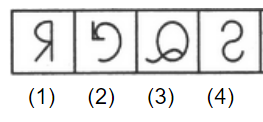a. 1 b. 2 c. 3 d. 4

 47. Choose the figure which is different from the rest.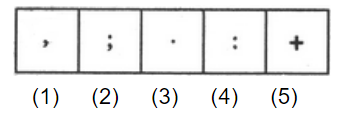a. 1 b. 2 c. both 3 and 4 d. 5
 48. Choose the figure which is different from the rest.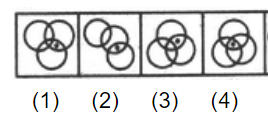a. 1 b. 2 c. 3 d. 4
 49. Choose the figure which is different from the rest.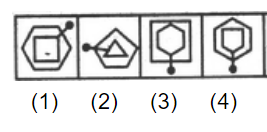a. 1 b. 2 c. 3 d. 4
 50. Choose the figure which is different from the rest.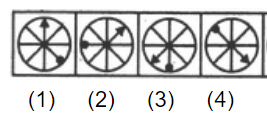a. 1 b. 2 c. 3 d. 4